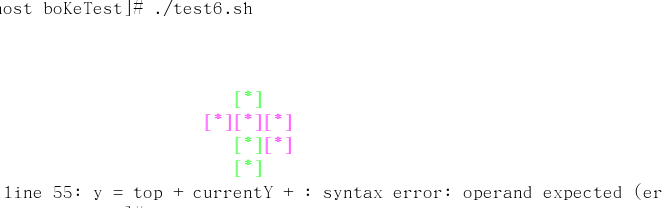1、为什么第55行老是报错，错误为：line 55: y = top + currentY + : syntax error: operand expected (error token is " ")

2、 DrawCurBox 0不能够将屏幕上的方块抹去喃？`````` #!/bin/bash

#将七种方块全部定义出来
#通过旋转，我们便可以得到很多种样式的方块
box0=(0 0 0 1 1 0 1 1)
box1=(0 2 1 2 2 2 3 2 1 0 1 1 1 2 1 3)
box2=(0 0 0 1 1 1 1 2 0 1 1 0 1 1 2 0)
box3=(0 1 0 2 1 0 1 1 0 0 1 0 1 1 2 1)
box4=(0 1 0 2 1 1 2 1 1 0 1 1 1 2 2 2 0 1 1 1 2 0 2 1 0 0 1 0 1 1 1 2)
box5=(0 1 1 1 2 1 2 2 1 0 1 1 1 2 2 0 0 0 0 1 1 1 2 1 0 2 1 0 1 1 1 2)
box6=(0 1 1 1 1 2 2 1 1 0 1 1 1 2 2 1 0 1 1 0 1 1 2 1 0 1 1 0 1 1 1 2)

#把所有有的方块的定义都放到box变量中
#相当于定义了一个二维数组
box=(\${box0[@]} \${box1[@]} \${box2[@]} \${box3[@]} \${box4[@]} \${box5[@]} \${box6[@]})
#各种方块旋转后可能得到的样式数目
rotateCount=(1 2 2 2 4 4 4)
#各种方块在box变量中的偏移量，即每个一维数组在二维数组中的偏移位置
boxOffset=(0 1 3 5 7 11 15)
#颜色数组，存放颜色的值，以便随机产生颜色用
#30为黑色，一般不使用
colourArray=(31 32 33 34 35 36 37)
#旋转角度，或者是旋转类型,初始值为－1
rotateType=-1
#当前所选择的盒子的标号
boxNum=-1
#存放当前随机后的颜色
colourNum=-1
#将某个盒子的某种旋转类型的方块的坐标点存放到newBox中
#存放的方块单一，方便使用
newBox=()

#边缘距离
left=3
top=3

#当前方块位置的坐标点
currentX=10
currentY=2

#画方块的函数
function DrawCurBox()
{
local i j k x y bDraw

bDraw=\$1
if (( bDraw == 0 ))
then
for ((i = 0; i < 8; i += 1))
do
((j = i + 1))
((x = left + 3 * (curentX + \${newBox[\$i]})))
((y = top + currentY + \${newBox[\$j]}))

echo -e "\033[\${y};\${x}H         "
done
else
#colourNum 是产生的随机数，高亮度
echo -e "\033[\${colourArray[\$colourNum]}m\033[1m"
for ((i = 0; i < 8; i += 2))
do
((k = i + 1))
((x = left + 3 * (currentX + \${newBox[\$i]})))
((y = top + currentY + \${newBox[\$k]}))

echo -e "\033[\${y};\${x}H[*]"
done
fi
echo -e "\033[0m"
}

#随机产生方块
function Random_Box()
{
local i j
#随机产生盒子
((boxNum = \$RANDOM % 7))
#盒子找到了，现在就是从某个黑字里面选择盒子的旋转类型，既那种类型的方块出来
((rotateType = \$RANDOM % \${rotateCount[\$boxNum]}))
#随机产生颜色
((colourNum = \$RANDOM % \${#colourArray[*]}))

#找到需要的方块在box中的起始位置
#乘以8的原因，此处的起始位置，只是一个逻辑位置，实际的位置是每一个
#位置都有八个坐标点，故需乘以八
for ((j = 0,i = (\${boxOffset[boxNum]} + \$rotateType) * 8; j < 8; j++,i++))
do
newBox[\$j]=\${box[\$i]}
done
}

while :
do
Random_Box
DrawCurBox 1
sleep 1
DrawCurBox 0
done

``````

1个回答

line 55: y = top + currentY + : syntax error: operand expected (error token is " ")

((y = top + currentY + \${newBox[\$j]}))